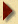6th Puzzle Answer

If we make ß = Pi/2 (+/- 2k Pi) radians and R = 1, then the second formula is equal to i.
Therefore i = ei(Pi/2 +/- 2k Pi)
Raising to the power 1/i we get: i(1/i) = e(Pi/2 +/- 2k Pi)
There is an infinity of answers and they are all real (Who said the god of mathematicians did not have a sense of humour?).
The first one, e(Pi/2) = 4.81048...Puzzle Page